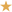Category: Maths and Statistics
Hits: 21240

User Rating: 4 / 5The Gibbs sampler has its origin in digital image processing and was introduced by Geman and Geman in 1984 for the Gibbs distribution. It was only in 1990 that Gelfand and Smith discovered that the Gibbs sampler works for other distributions as well.

The general idea of the Gibbs sampler is to approximate the modes and marginal distributions of an unknown distribution p(ω), where ω = (ω1,…,ωn), by successively taking samples from the known conditional distributions pi = p(ωij, j≠i).

The actual algorithm works as follows:

1. Set j = 0 and set initial values ω(0) = (ω1(0),…,ωn(0)).
2. For 1 ≤ i ≤ n obtain samples ωi(j) ∼ p(ω11(j),…,ωi−1(j)i+1(j−1),…,ωn(j−1)) from the conditional distributions and, therefore, a new value ω(j).
3. Increase j by 1 and continue with step 2.

Since the new values depend only on the immediately preceding ones, we clearly have a Markov process.

The Gibbs sampler was first used in digital image processing, an application we look at in sections 2 and 5.

In section 3 we will look at Markov random fields and Gibbs distributions and see how they are related to each other. The most important result in this section is that they are in fact equivalent.

We will see in section 4 that the Gibbs sampler converges to the unknown joint distribution p(ω), and we will introduce the process of annealing, that is, gradually reducing the temperature of the system to speed up convergence.

In section 6 we will generalise the results from section 4 and show that the Gibbs sampler works for other distributions as well. We will introduce two other related algorithms, the data-augmentation algorithm, which approximates the joint distribution given the conditional distributions, and the substitution sampling algorithm, which is very close related to the Gibbs sampler.

### Literature

MMath Project, University of York, 2001